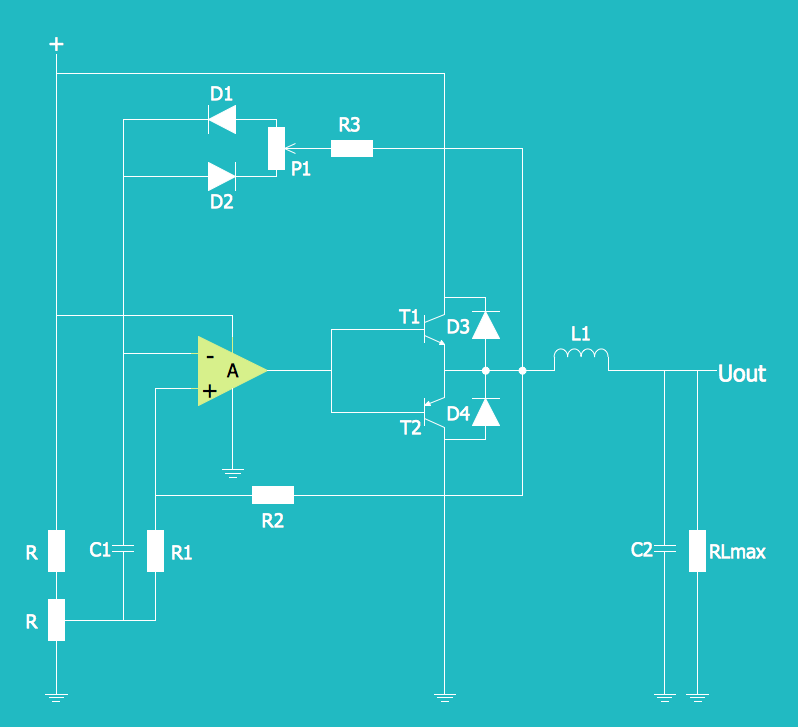This site uses cookies. By continuing to browse the ConceptDraw site you are agreeing to our Use of Site Cookies.
Engineering — Electrical

# Electrical Engineering

Samples of electronic schematics, digital and analog logic, circuit and wiring diagrams, power systems diagrams are created using ConceptDraw DIAGRAM diagramming and vector drawing software enhanced with Electrical Engineering solution from ConceptDraw Solution Park.

## Tutorials and Solutions:

Video Tutorials: ConceptDraw Solution Park

Solutions: Electrical Engineering for ConceptDraw DIAGRAM

## Sample 1: Circuit Diagram — Lamp

Electrical Engineering sample: Circuit diagram — lamp.

This example is created using ConceptDraw DIAGRAM diagramming software enhanced with Electrical Engineering solution from ConceptDraw Solution Park.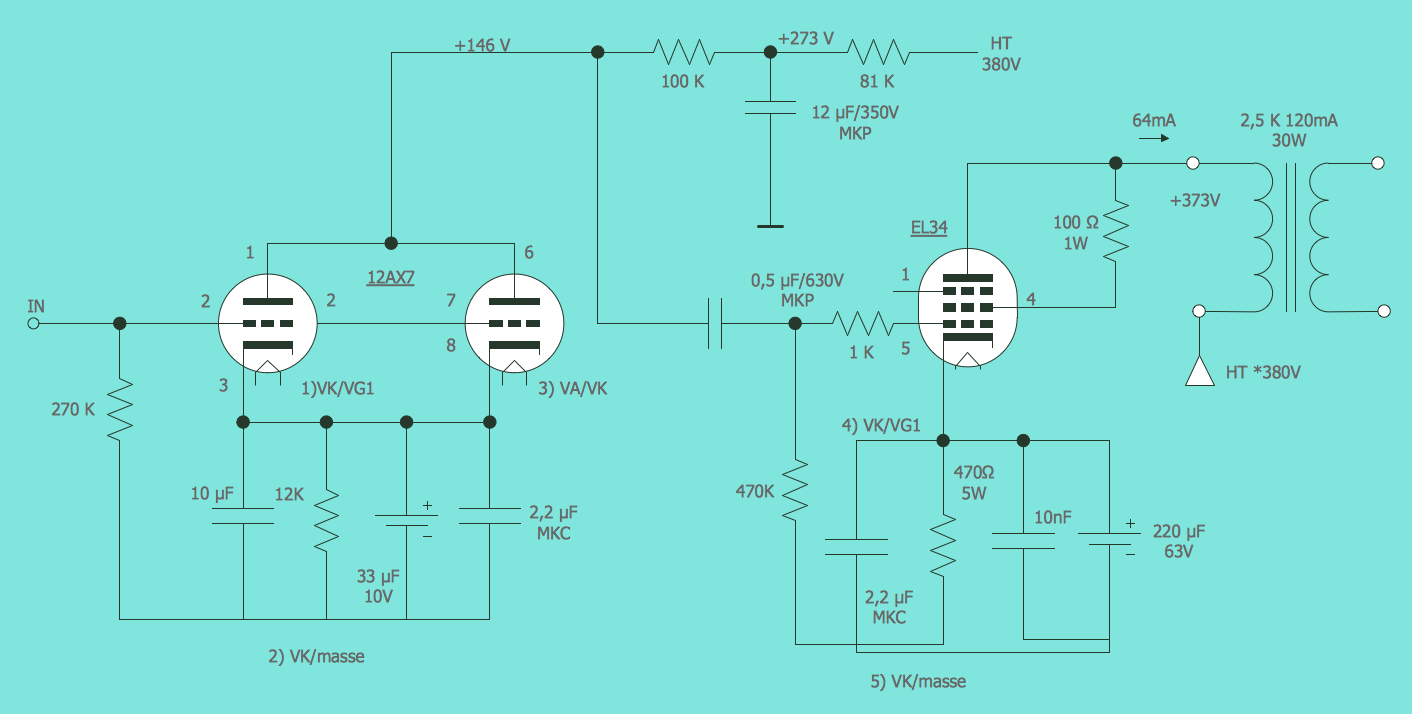## Sample 2: Electrical Diagram — 2 Bit ALU

Electrical Engineering sample: 2 bit ALU.

This example is created using ConceptDraw DIAGRAM diagramming software enhanced with Electrical Engineering solution from ConceptDraw Solution Park.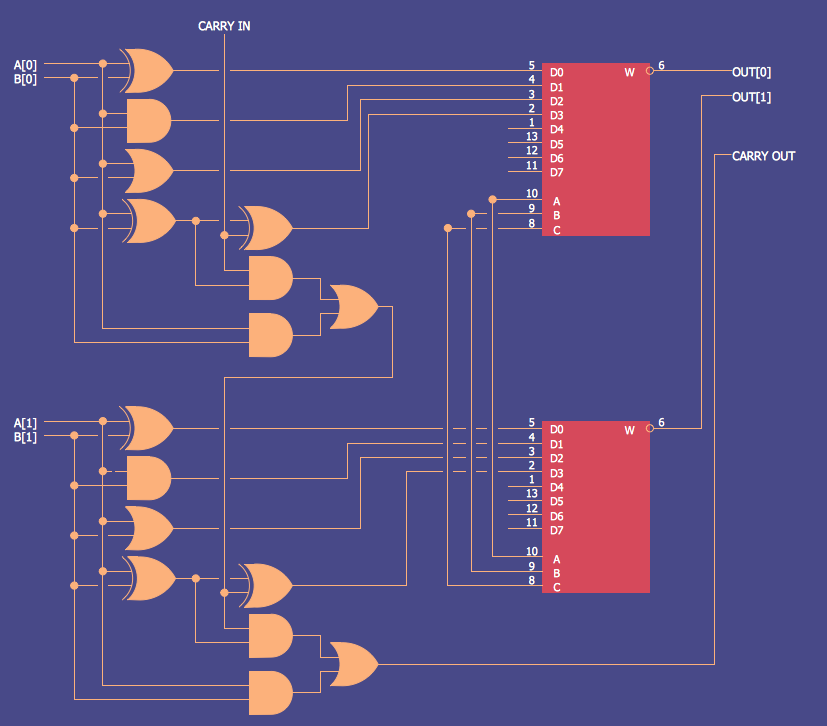## Sample 3: Electrical Schematic — Amplifier

Electrical Engineering sample: Amplifier.

This example is created using ConceptDraw DIAGRAM diagramming software enhanced with Electrical Engineering solution from ConceptDraw Solution Park.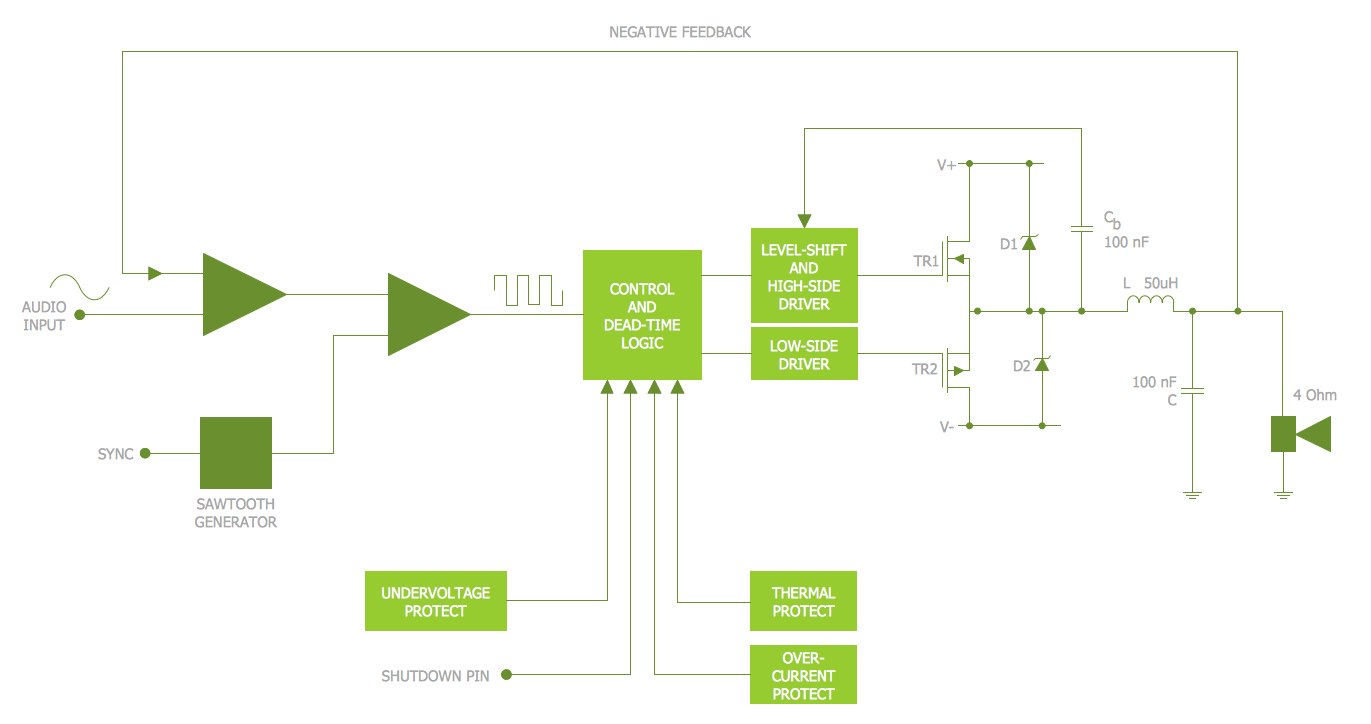## Sample 4: Electrical Diagram — Bipolar Current Mirror

Electrical Engineering sample: Bipolar current mirror.

This example is created using ConceptDraw DIAGRAM diagramming software enhanced with Electrical Engineering solution from ConceptDraw Solution Park.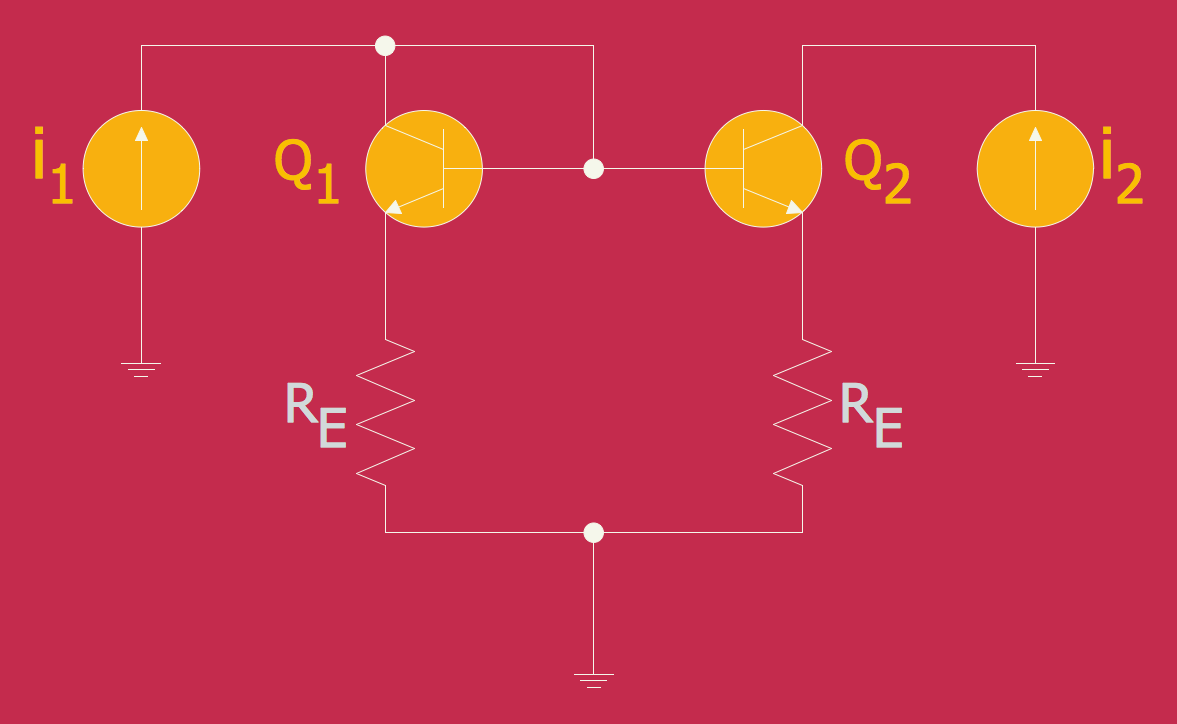## Sample 5: Electrical Diagram — Simple Switched Supply

Electrical Engineering sample: Simple switched supply.

This example is created using ConceptDraw DIAGRAM diagramming software enhanced with Electrical Engineering solution from ConceptDraw Solution Park.